[TOC]

# 1. Introduction to Data Compression

pb: reduce the size of a file, to save space/time for storing/transmitting.
applications: generic file compression(gzip), multimedia (mp3), communication(skype).

From binary data `B`, ⇒ generate a compressed representation `C(B)`.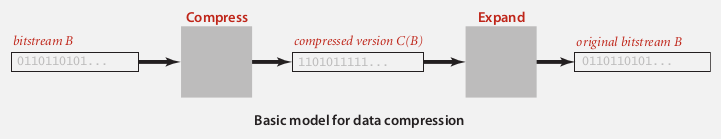lossless compression: get exactly `B` from `C(B)`
compression ratio: `|C(B)|/|B|`(||means number of bits)

### Tools

extension to stdio libraries: read/write bits

```public class BinaryStdIn{
boolean readBoolean(); // read 1 bit and return as a boolean
char readChar(); // read 8 bits and return a char
char readChar(int r); // read r (<=8) bits and return a char
// similar method for int/long/double
boolean isEmpty(); // is bitstream empty?
void close();
}

public class BinaryStdOut{
void write(boolean b); // write 1 bit
void write(char c); // write 8 bits
void write(char c, int r); // write r (least-significant) bits of c
// similar method for int/long/double
void close(); // might add some byte alignment bits
}
```

usage example: store a date 12/31/1999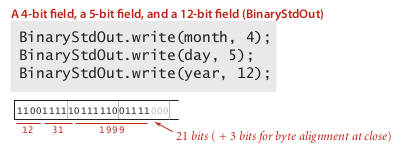universal date compression?
prop. NO algorithm can compress every bitstring.
pf. by contradiction: repeatedly compress the bitstring ⇒ bit length goes to 0.

# 2. Run-Length Coding

one simple type of redundancy in bitstream: long runs of repeated bits.
⇒ use 4-bit counts to represent alternating 1s and 0s.• question1: how many bits to represent counts ? ⇒ pick 8 bits (just tradeoff)
• question2: what if run length is bigger than max length(2^8)? ⇒ just add length=0 of the alternating bit...

application: JPEG...

code:

```public class RunLength{
private final static int R = 256; // max run length
private final static int lgR = 8;
public static void compress(){
boolean bit, oldbit = false;
int l=0;
while(!BinaryStdIn.isEmpty()){
bit = BinaryStdIn.readBoolean();
if(bit!=old){
oldbit = bit;
BinaryStdOut.write(l,lgR);
l = 0;
}
else if(l==R-1){
BinaryStdOut.write(l,lgR);
BinaryStdOut.write(0,lgR);
l = 0;
}
l ++;
}
BinaryStdOut.write(l,lgR);
BinaryStdOut.close();

}
public static void expand(){
boolean bit = false;
while(!BinaryStdIn.isEmpty()){
int l = BinaryStdIn.readInt(lgR);
for(int i=0;i<l;i++)
BinaryStdOut.write(bit);
bit != bit;
}
BinaryStdOut.close();
}
}
```

# 3. Huffman Compression

classique...

idea: variable length code.
ex. Morse code, more freq chars use less chars.
→ pb: ambiguity? one code is the prefix of another...
→ need to use prefix-free code

⇒ use a trie to represent the prefix-free code, in bitstream, use a binary trie: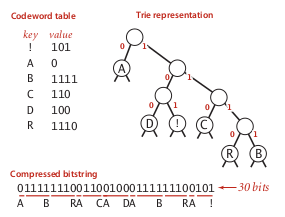### Huffman Trie Node

define trie nodes having frequences:

```public static class Node implements Comparable<Node>{
private char ch; // only used for leaves, null for internal nodes
private int freq;
private final Node left, right; // left-->0, right-->1
public Node(char ch, int freq, Node left, Node right){//...}
public boolean isLeaf(){
return left==null && right==null;}
public int compareTo(Node that){
return this.freq-that.freq;}
}
```

### implementation

expansion
in the bitstream, we first put the (serialized) trie in the head.

```public void expand{
Node root = getTrie(); //
int N = BinaryStdIn.readInt(); // number of chars in string
for(int i=0; i<N; i++){
Node x = root;
while (!x.isLeaf()){
if(BinaryStdIn.readBoolean()) x = x.right;
else x = x.left;
}
BinaryStdOut.write(x.ch);
}
BinaryStdOut.close();
}
```

output trie
ie. to serialize a trie.
⇒ use preorder traversal:
0 for internal nodes, and 1 for leaf (followed by the corresponding char)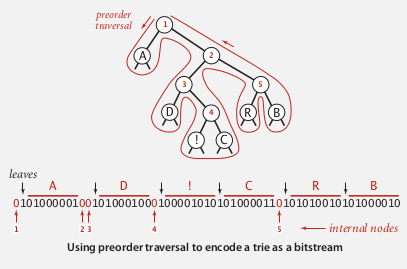recursive method:

```private static void writeTrie(Node x){
if(x==null) return;
if(x.isLeaf()){
BinaryStdOut.write(true); // leaf node
BinaryStdOut.write(x.ch); // followed by the char
} else {
BinaryStdOut.write(false); // internal node
writeTrie(x.left);
writeTrie(x.right);
}
}
```

read trie
Reconstruct the trie from the serialized bitstring.
preorder(x) = x+preorder(x.left)+preorder(x.right) ⇒ recursive method...

```private static Node readTrie(){
if(BinaryStdOut.readBoolean()) {
char ch = BinaryStdOut.readChar();
return new Node(ch, 0, null, null);
} else {
Node left = readTrie();
Node right = readTrie();
return new Node('\0', 0, left, right); // subtle recursion
}
}
```

### Huffman algorithm

pb: how to find the best prefixless code?

Shannon-Fano algo:

• partition symbols S into 2 subsets: S1, S0, with roughly equal freq
• code in S1 start with 1 and in S0 start with 0
• recur on S1 and S0

[Huffman algo] (1950)

• count letter freq in input
• build a node for each char
• select the 2 tries with minimum weight(freq), merge them, and put it back
• repeat until we get only 1 trie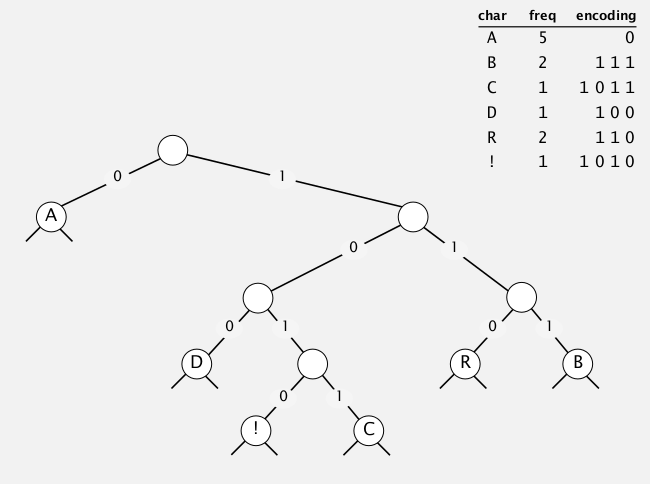application: jpeg, pdf, mp3, ...

implementation

```private static Node buildTrie(int[] freq){
MinPQ<Node> pq = new MinPQ<Node>();
for(char i=0; i<R; i++)
pq.insert( new Node(i, freq[i], null, null) );
while(pq.size()>1){
Node x = pq.pop(), y = pq.pop();
pq.insert( new Node('\0',x.freq+y.freq, x, y);
}
return pq.top();
}
```

prop. Huffman algorithm produces the optimal prefixless code.

running time: N + RlgR

# 4. LZW Compression

idea:
adaptive model: learn and update the model as you read the text.
decoder will do the same thing.

build a table of not only mapping chars to codes, but also mapping >=2-char combinations to codes(of fixed width).
when encoding strings, look for the longest prefix that is in our table.### LZW compression

LZW compression algo

• create a symbol table mapping string keys to W-bit code, initialize as mapping only chars to codes
• find longest prefix `s` of the unscanned input
• write out the corresponding code
• add `s+c` into the symbol table, where `c` is the next char in input

Use a trie for representing the code table → because support longest prefix match.

implementation

```public void compress(String input){
TST<Integer> st = new TST<Integer>(); // a trie symbol table
for(char i=0; i<R; i++)
st.put(""+(char)i, i);
int code = R+1; // R is used as "stop"
while(input.length()>0){
String s = st.longestPrefixOf(input);
BinaryStdOut.write(st.get(s), W);
int l = s.length();
if(l<input.length())
st.put(s+input.charAt(l), code++);
input = input.substring(l);
}
BinaryStdOut.write(R, W); // write "stop"
BinaryStdOut.close();

}
```

### LZW expansion

no need to get the codeword table, the input is just the compressed bitstring.

• maintain a (reverse)table mapping int(code) to strings.
• as we decode the string, we add new entries to our table.

to represent the table: just an array.

note when add new entries: add key = `s+c`, where `s` is the last decoded string, `c` is the first char of the currently decoded string.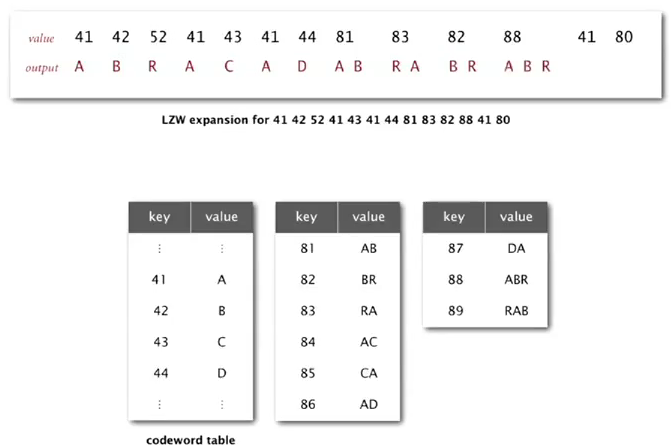example: when decoded ABR, s = BR, currently decoded string = ABR, so we add BRA.

tricky case: ABABABA
compression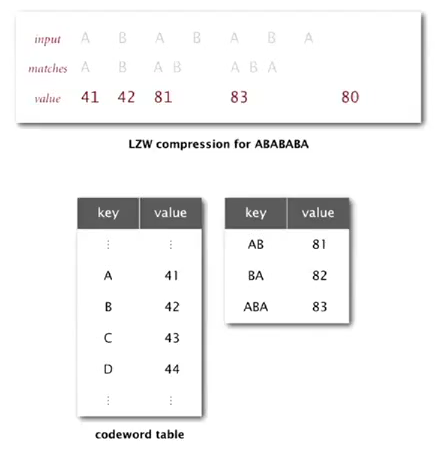problem in expansion when reading the "83":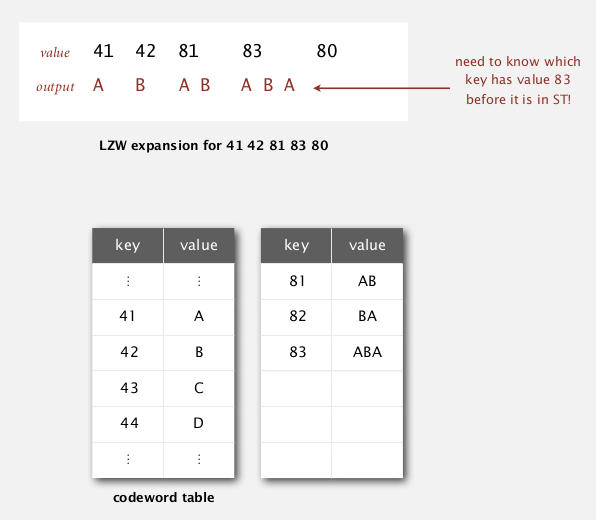→ need to be able to update the table when encountering a code not yet defined...

# Summary

• Huffman: fixed-length symbols, with variable-length codes.
• LZW: variable-length symbols, with fixed-length codes.

theoretical limit: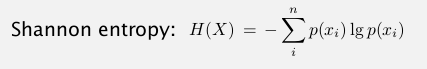#### Part 10 of series «Algorithms Princeton MOOC II»：

comments powered by Disqus